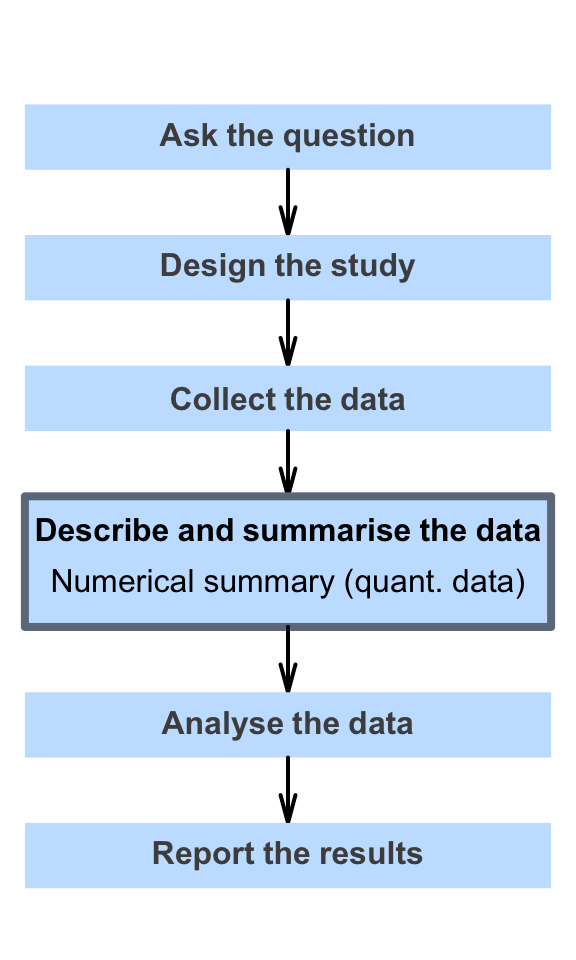# 13 Numerical summaries: quantitative data

So far, you have learnt to ask a RQ, identify different ways of obtaining data, design the study, collect the data describe the data, and graphically summarise data.

In this chapter, you will learn to numerically describe quantitative data. Both quantitative and qualitative data are described numerically in quantitative research. You will learn to:

• numerically summarise quantitative data using the appropriate statistics.
• describe quantitative data by average, variation, shape and unusual features.